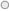# Effect of H2/CO Ratios on the Reduction Kinetics and Mechanism of Iron Ore Pellets

The H2/CO ratio in the reducing gas is one of the most important factors that affect the reduction rate of iron ore pellets in the direct reduction processes. The present study is focusing on the effect of H2/CO gas ratio on kinetics of direct reduction of iron ore pellets. The H2/CO ratio was in the range of 1.0-2.6 which simulates the reducing gas composition in different direct reduction technologies (Midrex, HyL, and Syngas based direct reduction). The reaction rate constants and the apparent activation energy of the reduction process were calculated for both of the experimental and mathematical regression model. The unreacted core shrinkage mathematical formulations are applied to determine the rate controlling mechanism. The highest regressions and the lowest deviations from straight lines were obtained by the application of the mathematical formulations that corresponded to the interfacial chemical reaction mechanism and mixed control of chemical reaction with gaseous diffusion mechanism. The comparison between the calculated apparent activation energy and the standard ranges indicated that the rate controlling mechanism is mixed of the interfacial chemical reaction and gaseous diffusion. The contribution of chemical reaction in the rate controlling mechanism increased as the H2/CO ratio increased. The reaction rate constants and the apparent activation energy values were found to increase as H2/CO ratio increased due to the higher diffusivity of H2 compared to that of CO gas.